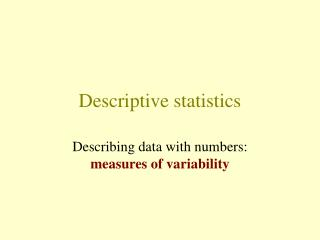DownloadDownload PresentationDescriptive statistics

# Descriptive statistics

Télécharger la présentation## Descriptive statistics

- - - - - - - - - - - - - - - - - - - - - - - - - - - E N D - - - - - - - - - - - - - - - - - - - - - - - - - - -
##### Presentation Transcript

1. Descriptive statistics Describing data with numbers: measures of variability

2. What to describe? • What is the “location” or “center” of the data? • How do the datavary?

3. Measures of Variability • Range • Interquartile range • Variance and standard deviation • Coefficient of variation All of these measures are appropriate for measurement data only.

4. Range • The difference between largest and smallest data point. • Highly affected by outliers. • Best for symmetric data with no outliers.

5. What is the range?

6. Range Descriptive Statistics Variable N Mean Median TrMean StDev SE Mean GPA 92 3.0698 3.1200 3.0766 0.4851 0.0506 Variable MinimumMaximum Q1 Q3 GPA 2.02003.9800 2.6725 3.4675 Range = 3.98 - 2.02 = 1.96

7. Interquartile range • The difference between the “third quartile” (75th percentile) and the “first quartile” (25th percentile). So, the “middle-half” of the values. • IQR = Q3-Q1 • Robust to outliers or extreme observations. • Works well for skewed data.

8. What is the Interquartile Range?

9. Interquartile range Descriptive Statistics Variable N Mean Median TrMean StDev SE Mean GPA 92 3.0698 3.1200 3.0766 0.4851 0.0506 Variable Minimum Maximum Q1Q3 GPA 2.0200 3.9800 2.67253.4675 IQR = 3.4675 - 2.6725 = 0.795

10. Variance 1. Find difference between each data point and mean. 2. Square the differences, and add them up. 3. Divide by one less than the number of data points.

11. Variance • If measuring variance of population, denoted by 2 (“sigma-squared”). • If measuring variance of sample, denoted by s2 (“s-squared”). • Measures average squared deviation of data points from their mean. • Highly affected by outliers. Best for symmetric data. • Problem is units are squared.

12. Standard deviation • Sample standard deviation is square root of sample variance, and so is denoted by s. • Units are the original units. • Measures average deviation of data points from their mean. • Also, highly affected by outliers.

13. Variance or standard deviation Sex N Mean Median TrMean StDev SE Mean female 126 91.23 90.00 90.83 11.32 1.01 male 100 06.79 110.00 105.62 17.39 1.74 Minimum Maximum Q1 Q3 female 65.00 120.00 85.00 98.25 male 75.00 162.00 95.00 118.75 Females: s = 11.32 mph and s2 = 11.322 = 128.1 mph2 Males: s = 17.39 mph and s2 = 17.392 = 302.5 mph2

14. What is the variance or standard deviation?

15. Variance or standard deviation Sex N Mean Median TrMean StDev SE Mean female 126 152.05 150.00 151.39 18.86 1.68 male 100 177.98 183.33 176.04 28.98 2.90 Sex Minimum Maximum Q1 Q3 female 108.33 200.00 141.67 163.75 male 125.00 270.00 158.33 197.92 Females: s = 18.86 kph and s2 = 18.862 = 355.7 kph2 Males: s = 28.98 kph and s2 = 28.982 = 839.8 kph2

16. Coefficient of Variation • Ratio of sample standard deviation to sample mean multiplied by 100. • Measures relative variability, that is, variability relative to the magnitude of the data. • Unitless, so good for comparing variation between two groups.

17. Coefficient of variation (MPH) Sex N Mean Median TrMean StDev SE Mean female 126 91.23 90.00 90.83 11.32 1.01 male 100 106.79 110.00 105.62 17.39 1.74 Minimum Maximum Q1 Q3 female 65.00 120.00 85.00 98.25 male 75.00 162.00 95.00 118.75 Females: CV = (11.32/91.23) x 100 = 12.4 Males: CV = (17.39/106.79) x 100 = 16.3

18. Coefficient of variation (KPH) Sex N Mean Median TrMean StDev SE Mean female 126 152.05 150.00 151.39 18.86 1.68 male 100 177.98 183.33 176.04 28.98 2.90 Sex Minimum Maximum Q1 Q3 female 108.33 200.00 141.67 163.75 male 125.00 270.00 158.33 197.92 Females: CV = (18.86/152.05) x 100 = 12.4 Males: CV = (28.98/177.98) x 100 = 16.3

19. The most appropriate measure of variability depends on … the shape of the data’s distribution.

20. Choosing Appropriate Measure of Variability • If data are symmetric, with no serious outliers, use range and standard deviation. • If data are skewed, and/or have serious outliers, use IQR. • If comparing variation across two data sets, use coefficient of variation.